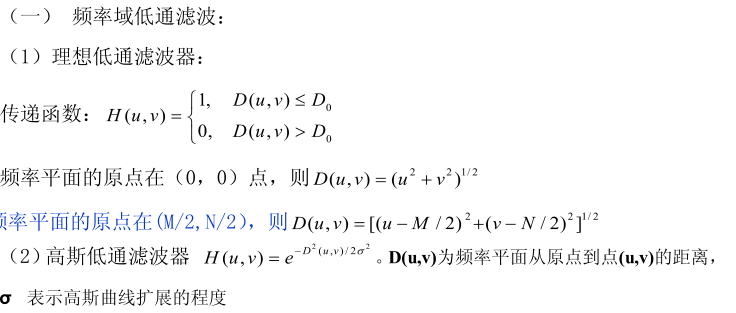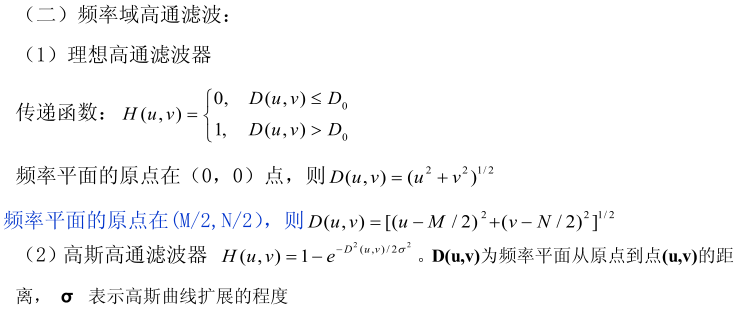# 图像处理复习

《图像处理》复习内容

1.图像传感器

2.数字化扫描

3.光电转换设备

3.合成图像

#### 5、图像的采样和量化

1.采样：将图像在空间（x,y)上离散化称为采样

2.量化：将采样后的图像离散为像素

#### 7、傅里叶变换

$F(u) = \int_{- \infty}^{+\infty}f(x)e^{-j 2 \pi u x}dx$

$f(x) = \int_{-\infty}^{+\infty}F(u)e^{j2\pi ux}du$

$F(u) = \int_{- \infty}^{+\infty}\int_{- \infty}^{+\infty}f(x)e^{-j 2 \pi (ux+uy)}dx$

$f(x) = \int_{- \infty}^{+\infty}\int_{- \infty}^{+\infty}F(u)e^{-j 2 \pi (ux+uy)}du$

$F(u)=\sum_{x=0}^{N-1}f(x)e^{-j\frac{2\pi ux}{N}}$

$f(x)=\frac{1}{N}\sum_{u=0}^{N-1}F(u)e^{-j\frac{2\pi ux}{N}}$

1.线性特性 2.比例特性 3.平移性质 4.可分离性 5.周期性 6.共轭对称性 7.旋转不变性 8.微分性 9平均值性质 10.卷积定理 11.相关定理 12.帕沙瓦（Parseval)定理（能量不变)

$F(u,v)=\sum_{x=0}^{M-1}\sum_{y=1}^{N-1}f(x,y)e^{-j2\pi(\frac{ux}{M}+\frac{vy}{N})}$

$f(x,y)=\frac{1}{MN}\sum_{u=0}^{M-1}\sum_{v=1}^{N-1}F(u,v)e^{-j2\pi(\frac{ux}{M}+\frac{vy}{N})}$

#### 8、离散余弦变换

DFT(离散傅里叶变换)是频谱分析的有力工具，但DFT是基于复数域的运算，因而给实数运算带来不变。

DCT变换具有正交变换的性质1）空域增强

1.1.灰度变换

1.1.1直接灰度变换

1.1.2直方图灰度变换

1.1.3图像代数运算

1.2.空域滤波

1.2.1平滑滤波

1.2.2锐化滤波

2）频域增强

2.1 低频滤波

2.2 高通滤波

2.3 带通滤波

2.4 带阻滤波

#### 11、直方图的图像增强

1.首先对原始图像进行直方图均衡化处理

2.依据原始图像均衡化后的图像的灰度值得到目标图像的灰度级z

12、空域滤波

13、低通滤波#### 14、高通滤波16、无约束复原技术

17、逆滤波器

18、几何畸变校正

19、盲目图像复原

1）直接测量法 2)间接估计法

20、图像编码的基本原理

21、图像统计编码

22、预测编码

23、图像分割的基本概念

24、阀值分割

25、区域分割

26、边缘检测

27、几何特征

28、颜色特征

29、纹理特征

#### 30、骨架的概念©️2019 CSDN 皮肤主题: 精致技术 设计师: CSDN官方博客